# ofdmChannelResponse

Calculate OFDM channel response

Since R2023a

## Syntax

``hest = ofdmChannelResponse(pathgains,pathfilters,nfft,cplen)``
``hest = ofdmChannelResponse(pathgains,pathfilters,nfft,cplen,activesc)``
``hest = ofdmChannelResponse(pathgains,pathfilters,nfft,cplen,activesc,toffset)``

## Description

````hest = ofdmChannelResponse(pathgains,pathfilters,nfft,cplen)` returns the frequency response of a time-varying channel for OFDM signals with FFT length equal to `nfft` and cyclic prefix length equal to `cplen`. The channel is specified by `pathgains` and `pathfilters`. For more information, see OFDM Channel Response.```
````hest = ofdmChannelResponse(pathgains,pathfilters,nfft,cplen,activesc)` returns the frequency response of the channel for OFDM subcarriers specified by the `activesc` vector.```

example

````hest = ofdmChannelResponse(pathgains,pathfilters,nfft,cplen,activesc,toffset)` returns the frequency response of the channel for OFDM subcarriers specified by the `activesc` vector and the timing offset specified by `toffset`.```

## Examples

collapse all

Estimate the channel response for a beamformed OFDM signal with ${\mathit{N}}_{\mathrm{S}}$ parallel data streams filtered through an ${\mathit{N}}_{\mathrm{T}}$-by-${\mathit{N}}_{\mathrm{R}}$ MIMO channel. Use the channel response to apply OFDM equalization to the received OFDM-demodulated signal.

Define simulation variables.

```rng(1); numStreams = 2; % Number of parallel data streams (Ns) numTx = 4; % Number of transmit antennas (Nt) numRx = 3; % Number of receive antennas (Nr) bps = 6; % Bits per QAM symbol (and OFDM data subcarrier) nfft = 256; % FFT length cpLen = 16; % Cyclic prefix length numOFDMSym = 10; % Number of OFDM symbols SNRdB = 40; % Signal-to-noise ratio```

Check that the number of data streams is no greater than either the number of transmit antennas or the number of receive antennas.

```if numStreams > min(numTx,numRx) error('numStreams must be equal to or less than numTx and numRx.'); end```

Configure OFDM subcarriers.

```ofdmNullIdx = ... % Guard bands and DC subcarrier [1:9 (nfft/2+1) (nfft-8+1:nfft)]'; numDataSC = ... % Number of data subcarriers nfft-length(ofdmNullIdx);```

Generate an array of data symbols consisting of ${\mathit{N}}_{\mathrm{S}}$ parallel data streams, QAM-modulate the symbols, and then OFDM-modulate the QAM-modulated symbols.

```dataBits = randi([0,1],[numDataSC*bps numOFDMSym numStreams]); M = 2^bps; % Modulation order qamTx = qammod(dataBits,M, ... InputType="bit", ... UnitAveragePower=true); ofdmOut = ofdmmod(qamTx,nfft,cpLen,ofdmNullIdx);```

Beamforming expands the dimensionality of the transmit signal to improve link performance over multipath channels. The data streams are fed through a beamformer that focuses the transmit energy over an ${\mathit{N}}_{\mathrm{T}}×1\text{\hspace{0.17em}}$transmit antenna array where the number of antennas ${\mathit{N}}_{\mathrm{T}}\ge {\mathit{N}}_{\mathrm{S}}$.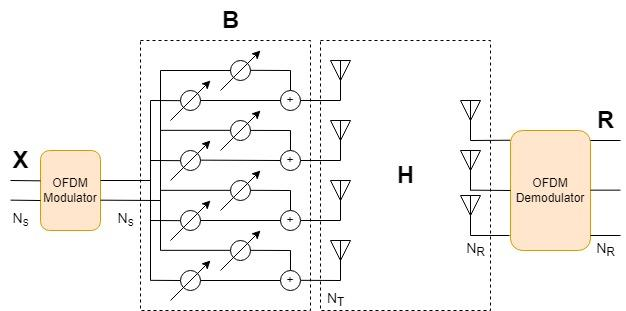Form a beamformer matrix from steering vectors acting on each stream.

```% Beamform the transmitted signal fc = 1e9; lambda = physconst('LightSpeed')/fc; beamAngles = 15; antIdx = (0:numTx-1); antDelay = 2*pi*sin(2*pi*beamAngles*(0:numStreams-1).'/360)/lambda; B = exp(1i*antIdx.*antDelay); % Ns x Nt beamformer matrix txOut = ofdmOut * B;```

Filter the OFDM-modulated signal through a MIMO channel to get the channel estimates.

```mimoChannel = comm.MIMOChannel( ... SampleRate=1e6, ... PathDelays=[0 3e-6 5e-6], ... AveragePathGains=[0 0.5 0.2], ... MaximumDopplerShift=0, ... SpatialCorrelationSpecification="None", ... NumTransmitAntennas=numTx, ... NumReceiveAntennas=numRx, ... PathGainsOutputPort=true); [channelOut,pathGains] = mimoChannel(txOut);```

In a practical system, the channel must be sounded to get the channel estimates. Instead of sounding, the `ofdmChannelResponse` function computes the exact channel estimates using the pathgains and pathfilters that are available after you pass data through the MIMO channel System object. Use channel path gains returned by the MIMO channel object, and the path filters and timing offset returned by the `info` object function, to estimate the OFDM channel response. If ${\mathit{N}}_{\mathrm{T}}<{\mathit{N}}_{\mathrm{R}}$, the channel forms an over-determined system (there are more receive antennas than necessary to adequately decode the transmitted signals). Call `ofdmChannelResponse` with the `pathGains` from the MIMO channel function to get the best channel estimates.

```mimoChannelInfo = info(mimoChannel); pathFilters = mimoChannelInfo.ChannelFilterCoefficients; toffset = mimoChannelInfo.ChannelFilterDelay; hest = ofdmChannelResponse(pathGains,pathFilters,nfft,cpLen, ... setdiff(1:nfft,ofdmNullIdx),toffset); % Nsc x Nsym x Nt x Nr [rxIn,nVar] = awgn(channelOut,SNRdB,"measured");```

Before demodulating the OFDM signal, account for the timing offset and symbol offset, remove the initial samples, and then pad with zeros to keep the signal length unchanged.

```zeropadding = zeros(toffset,numRx); ofdmDemodIn = [rxIn(toffset+1:end,:); zeropadding]; symOffset = cpLen/2;```

OFDM-demodulate and equalize the received signal.

`rxSym = ofdmdemod(ofdmDemodIn,nfft,cpLen,symOffset,ofdmNullIdx);`

The effects of beamforming and the MIMO channel affect the received data streams. The "effective" channel (${\mathit{H}}_{\mathrm{eff}}$) is an ${\mathit{N}}_{\mathrm{S}}$-by-${\mathit{N}}_{\mathrm{R}}$ matrix defined as the product of the transmit beamformer and the estimated MIMO channel.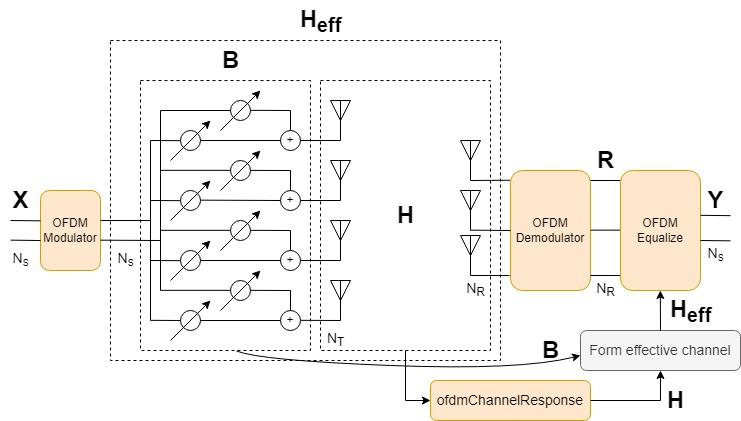To use the OFDM channel response when equalizing the OFDM-demodulated signal, you must reshape the ${\mathit{N}}_{\mathit{SC}}$-by-${\mathit{N}}_{\mathrm{OFDMsym}}$-by-${\mathit{N}}_{\mathrm{T}}$-by-${\mathit{N}}_{\mathrm{R}}$ array to an ${\mathit{N}}_{\mathrm{QAMsym}}$-by-${\mathit{N}}_{\mathrm{T}}$-by-${\mathit{N}}_{\mathrm{R}}$ array. Form the effective channel using the beamformer matrix `B` and the reshaped channel estimates `hest`. Equalize the received OFDM signal using the calculated effective channel, the noise variance, and the MMSE algorithm.

```hestReshaped = reshape(hest,[],numTx,numRx); heff = zeros(numDataSC*numOFDMSym,numStreams,numRx); for k = 1:numOFDMSym*numDataSC heff(k,:,:) = B * squeeze(hestReshaped(k,:,:)); end eqSym = ofdmEqualize(rxSym,heff,nVar);```

Show the received OFDM-demodulated symbols (`rxSym`) and the equalized OFDM-demodulated symbols (`eqSym`). The constellation of the OFDM-demodulated symbols before equalization does not resemble the QAM constellation. After equalization, the constellation points lay near the reference constellation.

```figure(1); scatterplot(rxSym(:));```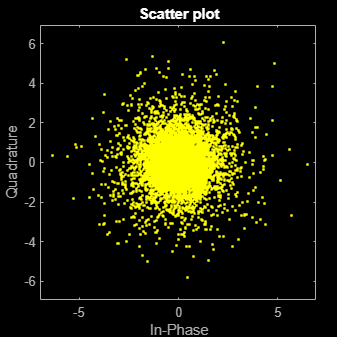```figure(2); scatterplot(eqSym(:));```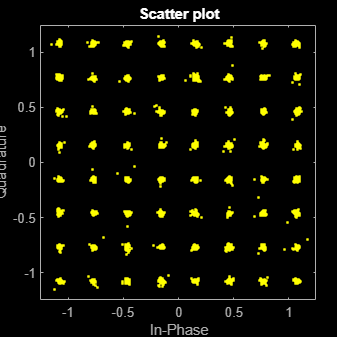## Input Arguments

collapse all

Channel path gains, specified as an NS-by-NP-by-T-by-NR array.

• NS is the number of samples.

• NP is the number of paths.

• NT is the number of transmit antennas.

• NR is the number of receive antennas.

The channel path gains follow the definition of channel path gains as calculated and output by the fading channel System objects. For example, see `comm.MIMOChannel`, `comm.RayleighChannel`, `comm.RicianChannel`, or `comm.ChannelFilter`.

Data Types: `double` | `single`
Complex Number Support: Yes

Channel path filter coefficients, specified as an NP-by-NH matrix.

• NP is the number of paths.

• NH is the number of impulse response samples.

The coefficient matrix is used to convert path gains to channel filter tap gains for each sample and each pair of transmit and receive antennas. The channel path filter coefficients follow the definition of channel path filter coefficients as calculated by the `info` object function of the fading channel System objects.

Data Types: `double` | `single`

FFT length, specified as a positive, integer scalar. The FFT length must align with that of the OFDM-modulated signal.

Data Types: `double` | `single`

Cyclic prefix length of one OFDM symbol, specified as a nonnegative, integer scalar with a value less than `nfft`.

Data Types: `double` | `single`

Number of active subcarriers, specified as a positive, integer scalar or column vector of values in the range [`1`, `nfft`].

Data Types: `double` | `single`

Timing offset in samples, specified as a nonnegative, integer scalar.

Data Types: `double` | `single`

## Output Arguments

collapse all

Frequency response, returned as an NSC-by-NSym-by-NT-by-NR array.

## Algorithms

collapse all

### OFDM Channel Response

The OFDM channel response algorithm uses an FFT to compute the channel estimates by using the path gains and path filter coefficients available after you pass data through a MIMO channel. Use channel path gains returned by the MIMO channel object, and the path filters and timing offset returned by the `info` object function, to estimate the OFDM channel response.

This figure shows one OFDM symbol in addition to the input channel impulse and the output channel impulse response. The algorithm ignores samples outside the OFDM symbol.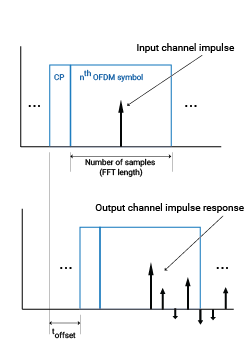For a time-varying channel, such as most fading channels, the impulse response depends on the location of the impulse at the channel input.

## Version History

Introduced in R2023a